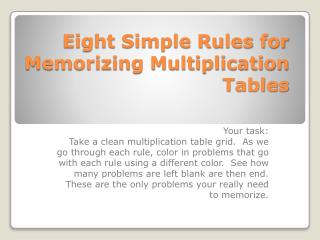# Eight Simple Rules for Memorizing Multiplication Tables - PowerPoint PPT PresentationDownload PresentationEight Simple Rules for Memorizing Multiplication Tables

Eight Simple Rules for Memorizing Multiplication TablesDownload Presentation## Eight Simple Rules for Memorizing Multiplication Tables

- - - - - - - - - - - - - - - - - - - - - - - - - - - E N D - - - - - - - - - - - - - - - - - - - - - - - - - - -
##### Presentation Transcript

1. Eight Simple Rules for Memorizing Multiplication Tables Your task: Take a clean multiplication table grid. As we go through each rule, color in problems that go with each rule using a different color. See how many problems are left blank are then end. These are the only problems your really need to memorize.

2. First Number Times Second Number is the Same as Second Number Times First Number • (also known as Commutative Property) • 2 x 3 = 3 x 2 • 4 x 6 = 6 x 4 • 7 x 8 = 8 x 7 • 9 x 5 = 5 x 9 Rule 1

3. Any Number Times One is that Number. • 2 x 1 = 2 • 3 x 1 = 3 • 4 x 1 = 4 • 5 x 1 = 5 • Ect….. Rule 2

4. To Multiply by Ten, Attach a Zero. • 1 x 10 = 100 • 2 x 10 = 200 • 3 x 10 = 300 • 4 x 10 = 400 • 5 x 10 = 500 • 6 x 10 = 600 Rule 3

5. To Multiply by Two, Double the Number • 2 x 2 = 2 + 2 • 4 • 3 x 2 = 3 + 3 • 6 • 5 x 2 = 5 + 5 • 10 • 7 x 2 = 7 + 7 • 14 • 9 x 2 = 9 + 9 • 18 • 12 x 2 = 12 + 12 • 24 Rule 4

6. Multiplying by Four is Doubling Twice • (Double-Double Rule) • 4 x 2= • 4 + 4 and 4 + 4 = 16 • 4 x 6 = • 6 + 6 and 6 + 6 = 24 • 4 x 3 = • 3 + 3 and 3 + 3 = 12 • 4 x 8 = • 8 + 8 and 8 + 8 = 32 Rule 5

7. Multiplying by Five is Just Counting by Fives • 1 x 5 = 5 • 2 x 5 = 10 • 3 x 5 = 15 • 4 x 5 = 20 • 5 x 5 = 25 • 6 x 5 = 30 • 7 x 5 = 35 • 8 x 5 = 40 • etc Rule 6

8. The Nine Rule – Tens Place is Number Minus One, Ones Place is Nine Minus Tens • Does anyone know any other patterns for learning nines? Rule 7

9. Now that you have colored all the “rules” in, how many blank problem are left? • Since there are patterns to help you remember all the other multiplication facts, these are the only problems you really need to memorize. Check your grid…now the last rule

10. Memorize the ten remaining facts • 3 x 3 = 9 • 3 x 6 = 18 • 3 x 7 = 21 • 3 x 8 = 24 • 6 x 6 = 36 • 6 x 7 = 42 • 6 x 8 = 48 • 7 x 7 = 49 • 7 x 8 = 56 • 8 x 8 = 64 Rule 8